or# Relations And Functions NCERT Solutions : Class 12 Maths

## NCERT Solutions for Class 12 Maths : Relations And FunctionsFilter
Filters :
Classes
•
•
•
•
•
•
•
Chapters
•
•
•
•
•
•
•
•
•
•
• 3 More
Exercises
•
•
•
•
•
•

### NCERT Class 12 | RELATIONS AND FUNCTIONS | Miscellaneous Exercise | Question No. 09

Given a non-empty set X, consider the binary operation `*: P(X)xx P(X) ->P(X)` given by `A" "*" "B" "=" "AnnB AAA ," "B" "in" "P(X)` is the power set of X. Show that X is the identity element for this operation and X is the only invertible element i### NCERT Class 12 | RELATIONS AND FUNCTIONS | Miscellaneous Exercise | Question No. 08

Given a non-empty set X, consider P(X) which is the set of all subsets of X. Define the relation R in P(X) as follows: For subsets A, B in P(X), ARB if and only if `A sub B`. Is R an equivalence relation on P(X)? Justify you answerDoubtnut one of the best online education platform provides free NCERT Solutions of Maths for Class 12 Relations And Functions which are solved by our maths experts as per the NCERT (CBSE) guidelines. We provide the solutions as video solutions in which concepts are also explained along with answer which will help students while learning , preparing for exams and in doing homework. These Solutions will help to revise complete syllabus and score more marks in examinations.

Get here free, accurate and comprehensive NCERT Solutions for Class 12 Maths Relations And Functions which have been reviewed by our maths counsellors as per the latest edition following up the CBSE guidelines. We provide video solutions in which solutions to all the questions of NCERT Class 12 Maths textbook are explained in step by step and detailed way.

## The Topics of the Chapter Relations And Functions are :

RELATION

TYPES OF RELATION

DIFFERENT TYPES OF MAPPINGS (FUNCTIONS)

COMPOSITE FUNCTION

INVERSE FUNCTION

TYPES OF BINARY OPERATIONS

IDENTITY ELEMENT

BINARY OPERATION

PROPERTIES OF COMPOSITE FUNCTIONS

DIFFERENT TYPES OF FUNCTIONS

PROPERTIES OF EQUIVALENCE RELATION

PROPERTIES OF INVERSE OF A FUNCTION

INVERSE OF AN ELEMENT

IDENTICAL FUNCTION

INTRODUCTION

It contains these exercises along with solved examples. All the exercises are solved in the video. Select the exercise to view the solutions exercisewise of the Chapter Relations And Functions:

## NCERT Solutions Class 12 Maths Chapter Relations And Functions Exercises:

We have covered all the exercises and also Solved examples in the videos. Along with the practise exercise Students should also practise solved examples to clear the concepts of the Relations And Functions. If incase you have any doubt you can watch the solutions for the given questions.Watch the solutions for the given questions in which it is also explained in the video steps to solve the questions along with answers.

# NCERT Class 12 Solutions Chapter 1 Relations and Functions

Basically, a relation is a set or collection of ordered pairs. An ordered pair is a collection of inputs and outputs; it represents a relationship between the two numerical figures. Therefore, a relation is a set of inputs and outputs, and a function is a relation with one outcome for each input. All functions are relations; however, all relations are not functions.

Class 12 is a very crucial stage in every student's life as they have to appear for boards and they also have to prepare for their upcoming competitive exams; therefore, most of the students prefer NCERT book as it combines and covers the CBSE syllabus in an easy language. This is owing to the reason that NCERT books provide an easy explanation to even the difficult concepts giving various examples related to real life. The systematic approach followed to solve the problems is the highlight of the textbook and it makes the process of study easy for the learners.

NCERT books cover all that is required to face not just the CBSE board exams, but also aids in cracking the other competitive entrance examinations. The more you practice the more proficient you become in maths. For a subject like maths, it is essential that the learners practice more and more problems in order to learn the concepts of a chapter comprehensively.

At Doubtnut, an online tutorial platform, we provide all the solutions worked out for the NCERT textbook, exercise-wise. NCERT Class 12 Solutions Chapter 1 Relations and Functions are presented in the form of videos that can be accessed from anywhere and at any time. The students can rely on these solution videos as all problems, including the complex ones are broken down into simpler solutions by our experts who are available to solve students queries whenever students are in doubt. Theory, as well as exercises of the chapter, is available at our website and if students download the Doubtnut app it becomes easier and convenient for them to pass the examination with flying colors.

## Relations and Functions – Class 12 Maths NCERT Solutions

In previous chapters, students have learned about relation and functions and their application.  Now further in this chapter students will be learning about different types of relations and functions, the composition of functions, invertible functions, and binary operations. Let us recall and study the chapter in detail now.

### What are relations?

The term ‘relation’ in mathematics has been taken from the meaning of relation in English, according to which it shows how two objects or quantities are related with each other or have some recognizable connection or link between them or quantities. Let us consider A as the set of students of Class 12 of a school and B as the set of students of Class 11 of the same school.

Then kind of relations from A to B are:

(i) {(a, b) Ô‘ A × B: a is the brother of b},

(ii) {(a, b) Ô‘ A × B: a is the sister of b},

(iii) {(a, b) Ô‘ A × B: age of a is greater than the age of b},

However, mathematically we can define a relation R from A to B as an arbitrary subset of A × B.

If (a, b) Ô‘ R, we say that a is related to b under the relation R and we can write as a R b. In general,

(a, b) Ô‘ R, it is not much important whether there is a recognizable connection or link between a and b. As seen in Class XI, functions are a special kind of relations.

## Topics and Subtopics of NCERT Class 12 Maths Chapter 1 – Relations and functions

The chapter relations and functions is divided into further topics and subtopics in which the content of previous knowledge of the student is given as well as the current syllabus is covered in the form of theory and exercises. Knowing the topics and subtopics of a given chapter helps the learners be aware of the whole content in a short form. The Knowledge of topics and subtopics help students to plan their studies accordingly in an organized manner. Let us look at the Topics and Subtopics of NCERT Class 12 Maths Chapter 1 – Relations and functions, according to which the solutions are worked out on the website:

### Types of Relations

Different types of relations are as follows –

#### Empty Relation

If no element of A is therein set A then relation R in a set is called empty relation.

Therefore, A is not related to any element of A, i.e., R = φ Ì A × A.

#### Universal Relation

If each element of A is related to every element of A, a relation R in a set A is called universal relation,  i.e., R = A × A.

Both the empty relation and the universal relation are sometimes called trivial relations.

Example 1 - Let us consider A as the set of all students of a boy’s school. It shows that the relation R in A is given by R = {(a, b): a is the sister of b} is the empty relation and R= {(a, b): the difference between heights of a and b is less than 3 meters} is called the universal relation.

An equivalence relation plays a significant role in Mathematics and is one of the most important relations.

To study about equivalence relation, we should have knowledge of three types of relations, which are named as reflexive relation, symmetric relation, and transitive relation.

Definition A relation R in a set A is called

Reflexive Relation

If every element of set A is related to itself, the relation is called a Reflexive Relation. For every a ∈ A, (a, a) ∈ R.

#### Symmetric Relation

A relation R on a set A is said to be symmetric if a=b and b=a i.e. both sides are equivalent (a, b) ∈ R then (b, a) ∈ R, for all a & b ∈ A.

#### Transitive Relation

A relation in a set A is said to be transitive if a is related to and b is related to c then a is also related to c, (a, b) ∈ R, (b, c) ∈ R, then (a, c) ∈ R, for all a, b, c ∈ A

A relation is called equivalence relation if it consists of Reflexive, Symmetric, and Transitive. For example, if we throw two separate dices A & B and then we note down all the possible outcomes.

Define a relation R= {(a, b): a ∈ A, b ∈ B}, we find that {(1, 1), (2, 2), …, (6, 6) ∈ R} (reflexive). If {(a, b) = (1, 2) ∈ R} then, {(b, a) = (2, 1) ∈ R} (symmetry). ). If {(a, b) = (1, 2) ∈ R} and {(b, c) = (2, 3) ∈ R} then {(a, c) = (1, 3) ∈ R} (transitive)

### Types of Functions

A function is defined as a special relation, which relates each element of set A with one and only one element of set B. Both the sets A and B must be a non-empty set. Every function has a particular output for a particular input. Hence, f: A → B is a function such that for a ∈ A there is a unique element b ∈ B such that (a, b) ∈ f

#### One to One Function

A function f: A → B is One to One if for each element of A there is a distinct element of B. It is also known as Injective. Consider if a1 ∈ A and a2 ∈ B, f is defined as f: A → B such that f (a1) = f (a2)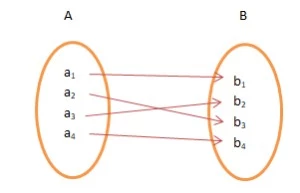#### Many to One Function

It is a function, which is related to two or more elements of A to the same element of set B. Two or more elements of A have the same relation in image B.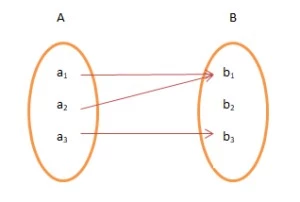#### Onto Function

A function f from A to B is called onto if for all b elements are in B there is an a element in A such that f(a) = b. That is, all elements in B are used.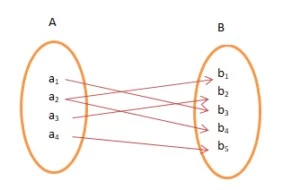#### One – One and Onto Function

A function, f is said to be One – One and onto or Bijective if the function f contains both One to One and onto function. In other words, the function f connects each element of A with a distinct element of B and every element of B has a pre-image in A.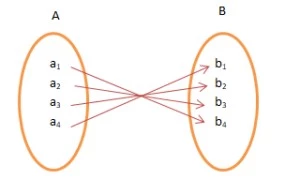### Other Types of Functions

In these functions, a set of all pairs of x and f(x) as coordinates are represented on the graph. But, let us get ready to know more about the types of functions and their graphs.

#### Identity Function

Let us take as the set of real numbers. Therefore if the function f: RR is defined as f(x) = y = x, for x ∈ R, then this type of function is known as Identity function. The domain and the range will be R. The graph will always be a straight line, which will pass through the origin.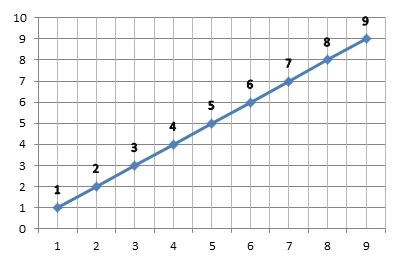Hence – f(x) = y = x

#### Constant Function

If this type of function f: RR which has c as a constant in R and is written as f(x) = y = c, for x ∈ R, then such function is known as Constant function. In this, the domain is R and its range is a constant, c. In this kind of graph, a straight line is obtained which is parallel to the x-axis.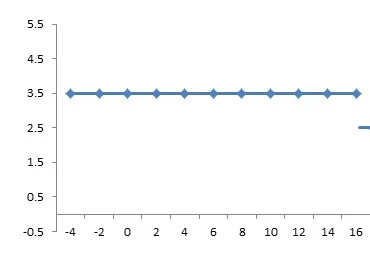Hence – f(x) = y = c =3.5

#### Polynomial Function

A polynomial function can be written as y =a+ a1x + a2x2 + … + anxn, where n is a non-negative integer and a0, a1, a2,…, ∈ R. The highest power in the expression is considered as the degree of the polynomial function. On the basis of their degree polynomial functions can be further divided as:

• Constant Function:  Expression which has degree zero, that polynomial function is called constant function
• Linear Function:  Polynomial function, which has a degree, one is called a linear function. Such as y = x + 1 or y = x or y = 2x – 5 etc. Taking y = x – 6. The domain and the range both are R. The graph obtained will always be a straight line.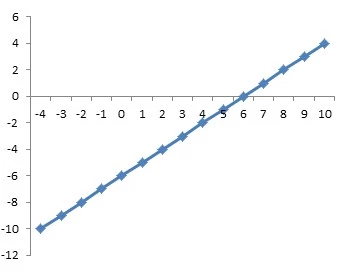Hence – f(x) = y = x-6

When degree of the polynomial function is two, then it is a known as quadratic function. In the expression f(x) = ax2 + bx + c, where a ≠ 0 and a, b, c are constant & x is a variable. The domain and the range are R. The graph represents, f(x) = x2 – 4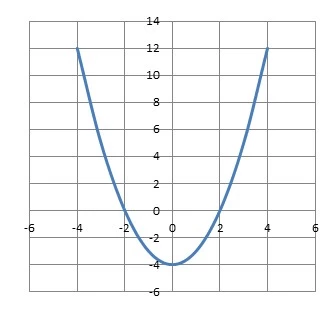• Cubic Function:  Polynomial function with degree three is said cubic function and can be denoted by f(x) = ax3 + bx2 + cx +d, where a ≠ 0 and a, b, c, and d are constant & x is a variable. Graph for f(x) = y = x3 – 5. The domain and the range is R
•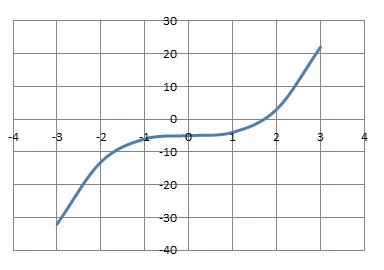Hence – f(x) = y = x3 - 5

#### Rational Function

A function which can be represented by a rational fraction such as, f(x)/g(x) in which numerator, f(x) and denominator, g(x) are polynomial functions of x, where g(x) ≠ 0. Let function f: R → R is termed say, f(x) = 1/(x + 2.5). The domain and the range are R. The Graph shows, the curves that seem to touch the axes-lines.

###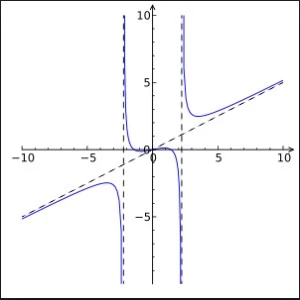#### Modulus Function

Any number having an absolute value, as c is represented in the form of |c|. If any function f: R→ R is defined by f(x) = |x|, it is termed as Modulus Function. For each non-negative value of x, f(x) = x and for each negative value of x, f(x) = -x i.e.,

f(x) = {x, if x ≥ 0; – x, if x < 0.

Its graph is shown below in which both the domain and the range are R.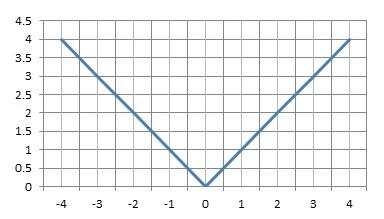Hence – f(x) = y = Ð†xÐ†

#### Greatest Integer Function

If a function f: R→ is defined by f(x) = [x], x ∈ X. In this, we take the round-off of the real number to the integer less than the number. Let the given interval is in the form of (k, k+1), the value of the greatest integer function is k, which is an integer. For example: [-21] = 21, [5.12] = 5. The graph represents -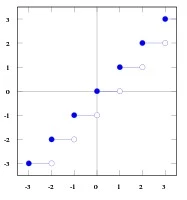#### Composition of Functions and Invertible Functions

Let f is a function, which relates A to B. In addition, there is another function g, which relates B to C. Can there be a relation between A to C? Therefore, we see that A is related to B and B is related to C and also A is related to C. Therefore, the indirect relation of elements of A to C is the basic concept of Composition of functions. In simple words combination of two functions in such a way that the output of one function becomes the input of others, the function is known as a composite function.

Stepwise application of the composition of functions is shown below. For example, the function f: A→ B & g: B→ C can be composed to form a function which relates x in A to g(f(x)) in C. All sets are non-empty sets. A composite function is denoted by (g o f) (x) = g (f(x)). The notation g o f is read as “g of f”.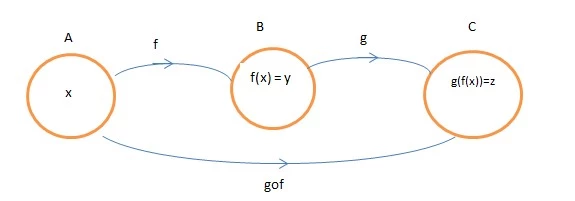Let us take the functions f: A→B and g: B→C. f = {1, 2, 3, 4, 5}→ {1, 4, 9, 16, 25} and g = {1, 4, 9, 16, 25} → {2, 8, 18, 32, 50}. A = {1, 2, 3, 4, 5}, B = {16, 4, 25, 1, 9}, C = {32, 18, 8, 50, 2}.Here, g o f = {(1, 2), (2, 8), (3, 18), (4, 32), (5, 50)}.

#### Invertible function

A function is said to be invertible if we reverse the order of mapping we get such input which acts as the new output. We can also say that, if a function, f whose domain is in set A and image in set B is invertible if f-1 has its domain in B and image in A.

f(x) = y ⇔ f-1 (y) = x.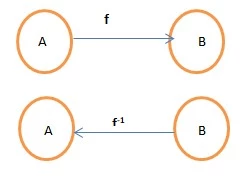It is not necessary all functions have an inverse. For a function to have an inverse, each element b∈ B must not have more than one a  A.  Also, each element of B must be mapped with that of A. The function must be a Surjective function. It is necessary that the function be of one-one and onto type to be invertible.

#### Binary Operations

The word binary means “two” so we can say that when we add or subtract, divide or multiply two numbers and obtain the third number as resultant. These operations (addition, subtraction, division, and multiplication) are called binary operations.

These are the calculations that combine two elements of the set to obtain another element of the same set. The binary operations on a non-empty set A are functions from A × A to A. The binary operation, *: A × A → A. These operations are of two elements of the set whose domains and co-domain are in the same set.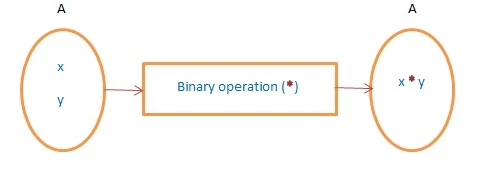## Significance of NCERT Mathematics Book Class 12

1. NCERT books are loaded with great study material. An ample number of exercises and examples are given of each and every topic which helps students to understand and learn the concepts in an easy and convenient language.
2. Numbers of examples are given based on daily life activities in NCERT books, which help students to understand the concept and definitions in an easy way.
3. The book contains an in-depth explanation of all the important concepts, which help students to understand the concepts in a better way.

The students are benefitted while practicing from the NCERT book and also if they have further doubts it can be cleared with the help of online tutorials videos which are given on our website where the experts are available to help them in every possible way. It might sound difficult in the beginning, but with the help of right study medium and materials, the students can easily master even the complex concepts. On our website, all of these concepts are taught in video format and this helps the students to learn the lessons with better concentration. The lessons taught in a step-by-step manner by an expert teacher helps in understanding the concepts in a better way. All the doubts occurring during the learning process can be easily clarified by just posting the picture of the question on the website. Thus, Doubtnut is the right platform and students should download the doubtnut app for easy access to their queries. Our website makes the perfect tutorial platform for the students to face both board exams and the competitive exams in a stress-free way!